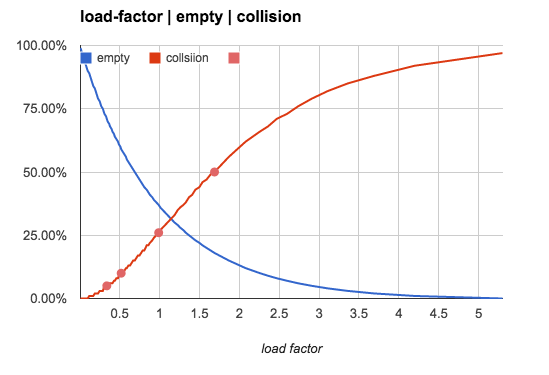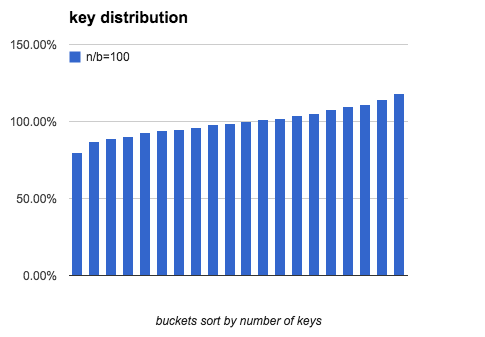# hash表中key的分布规律

## 当hash表中key和bucket数量一样时(n/b=1):

• 37% 的桶是空的.
• 37% 的桶里只有1个key.
• 26% 的桶里有1个以上的key(hash冲突).## key的数量对3类bucket数量的影响

n/b: (每个bucket平均key的数量) 空bucket占比 1个key的bucket占比 冲突占比
n/b=0.5 61% 30% 9%
n/b=0.75 47% 35% 17%
n/b=1.0 37% 37% 26%
n/b=2.0 14% 27% 59%
n/b=5.0 01% 03% 96%
n/b=10.0 00% 00% 100%## key的数量对bucket的均匀程度的影响

n/b超过1的时候(1个bucket允许存储多个key), 我们主要观察的对象就转变成bucket里key的数量的分布规律.

n/b: (bucket平均key的数量) 最少key的bucket的key的数量 最大差异(most-fewest)/most
1 0 100.0%
10 2 88.0%
100 74 41.2%
1,000 916 15.5%
10,000 9,735 5.1%
100,000 99,161 1.6%
1,000,000 997,996 0.4%

# Load Factor: n/b<0.75

hash表中常用一个概念 load factor $\alpha = \frac{n}{b}$. 来描述hash表的特征.

n/b=0.75 这个场景中, 如果不使用线性探测 (譬如使用bucket内的链表来保存多个的key), 大约有47% 的bucket是空的. 如果使用线性探测, 这47%中的 bucket, 有大约1半的的bucket 会被线性探测填充.

hash表特性小贴士:

• hash表本身是1个通过1定的空间浪费来换取效率的算法. 这三者不可同时兼得: 低时间开销(O(1)), 低空间浪费, 低冲突率.

• hash表只适合纯内存数据结构的存储:

• 必须很快, 因为hash表实际上是浪费空间换取了访问速度. 很多时候对磁盘的空间浪费是不能忍受的, 对内存的少许浪费是可以接受的.

• hash表只适合随机访问快的存储介质. 硬盘上的数据存储更多使用btree或其他有序的数据结构.

• 多数高级语言(内建了hash table/hash set等), 都保持 n/b<=0.75.

• hash表在n/b比较小的时候, 不会均匀的分配key!

# Load Factor: n/b>1

## n/b 越大, key的分布越均匀.

n/b 非常大的时候, 一个bucket是空的概率趋近于0, 而每个bucket中的key的数量趋于平均.

${avg} = \frac{n}{b}$b \ n 102 103 104 105 106
100 37.4% 13.6% 4.5% 1.4% 0.5%
1000 47.3% 17.7% 6.0% 1.9% 0.6%
10000 54.0% 20.9% 7.1% 2.3% 0.7%

key的数量(n) 确定时 bucket越多越不均匀.
bucket的数量(b) 确定时 key越多越均匀.
bucket和key的数量比例(n/b)一致时 n和b越大越均匀.

# 计算

## 每类bucket的数量

bucket的类型 bucket数量

### 空bucket 数量

$\lim_{b\to \infty} (1+\frac{1}{b})^b = e$.

$(\frac{b-1}{b})^n = ((1- \frac{1}b)^b)^{\frac{n}{b}} = e^{-\frac{n}{b}}$

$b e^{-\frac{n}{b}}$

### 有1个key的bucket的数量

\begin{align} & {n \choose 1} ( \frac{1}{b} )^1 ( 1 - \frac{1}{b} )^{n-1} \\ = & \frac{n}{b} e^{-\frac{n}{b}} \end{align}

$b\frac{n}{b} e^{-\frac{n}{b}} = n e^{ - \frac{n}{b} }$

### 多个key的bucket

$b - b e^{-\frac{n}{b}} - n e^{ - \frac{n}{b} }$

## key在bucket中分布的均匀程度

$p(i) = {n \choose i} ( \frac{1}{b} )^{ i } ( 1 - \frac{1}{b} )^{n-i}$

### 通过正太正态分布来近似

n, b 都很大时, 二项式分布 可以用正态分布正态分布来近似, 来估计key分布的均匀性:

\begin{align} p(i) = & {n \choose i}p^i(1-p)^{n-i} \\ \approx & \frac{1} {\sigma \sqrt{2 \pi} } e^{ - \frac{(i-\mu)^2} {2 \sigma^2} } \end{align} \begin{align} \mu = & np \\ \sigma^2 = & np(1-p) \\ \end{align}

1个bucket中key的数量不多于x的概率是:

$P(x) = \sum_{i=0}^x p(i)$

$b \cdot P(x) = b \cdot \sum_{i=0}^x p(i)$

### 计算最小key数量 x

$\sum_{i=0}^x p(i) \approx \int_{0}^{x} p(i) di \approx \int_{-\infty}^{x} p(i) di = \Phi( \frac{x-\mu}\sigma )$

$\Phi(x)$ 是正态分布的累计分布函数, 当x-u 趋近于0的时候, 可以使用以下方式来近似:

$\Phi(x)\; =\;0.5+\frac{1}{\sqrt{2\pi}}\cdot e^{-x^2/2}\left[x+\frac{x^3}{3}+\frac{x^5}{3\cdot 5}+\cdots+\frac{x^{2n+1}}{(2n+1)!!} + \cdots\right]$

$b \cdot \Phi(\frac{x-\mu}\sigma) = 1$

$\frac{u+(u-x)-x}{u+(u-x)} = 1 - \frac{x}{2u-x}$

u是均值n/b.

### 程序模拟

import sys
import math
import time
import hashlib

def normal_pdf(x, mu, sigma):
x = float(x)
mu = float(mu)

m = 1.0 / math.sqrt( 2 * math.pi ) / sigma
n = math.exp(-(x-mu)**2 / (2*sigma*sigma))

return m * n

def normal_cdf(x, mu, sigma):
# integral(-oo,x)

x = float(x)
mu = float(mu)
sigma = float(sigma)

# to standard form
x = (x - mu) / sigma

s = x
v = x
for i in range(1, 100):
v = v * x * x / (2*i+1)
s += v

return 0.5 + s/(2*math.pi)**0.5 * math.e ** (-x*x/2)

def difference(nbucket, nkey):

nbucket, nkey= int(nbucket), int(nkey)

# binomial distribution approximation by normal distribution
# find the bucket with minimal keys.
#
# the probability that a bucket has exactly i keys is:
#   # probability density function
#   normal_pdf(i, mu, sigma)
#
# the probability that a bucket has 0 ~ i keys is:
#   # cumulative distribution function
#   normal_cdf(i, mu, sigma)
#
# if the probability that a bucket has 0 ~ i keys is greater than 1/nbucket, we
# say there will be a bucket in hash table has:
# (i_0*p_0 + i_1*p_1 + ...)/(p_0 + p_1 + ..) keys.
p = 1.0 / nbucket
mu = nkey * p
sigma = math.sqrt(nkey * p * (1-p))

target = 1.0 / nbucket
minimal = mu
while True:

xx = normal_cdf(minimal, mu, sigma)
if abs(xx-target) < target/10:
break

minimal -= 1

return minimal, (mu-minimal) * 2 / (mu + (mu - minimal))

def difference_simulation(nbucket, nkey):

t = str(time.time())
nbucket, nkey= int(nbucket), int(nkey)

buckets =  * nbucket

for i in range(nkey):
hsh = hashlib.sha1(t + str(i)).digest()
buckets[hash(hsh) % nbucket] += 1

buckets.sort()
nmin, mmax = buckets, buckets[-1]

return nmin, float(mmax - nmin) / mmax

if __name__ == "__main__":

nbucket, nkey= sys.argv[1:]

minimal, rate = difference(nbucket, nkey)
print 'by normal distribution:'
print '     min_bucket:', minimal
print '     difference:', rate

minimal, rate = difference_simulation(nbucket, nkey)
print 'by simulation:'
print '     min_bucket:', minimal
print '     difference:', rate